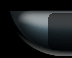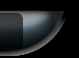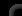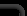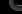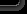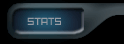76725 Posts in 13479 Topics by 1751 Members - Latest Member: LucBE1947 February 05, 2023, 05:13:58 am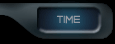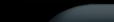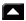|Home|Help|Search|Login|Register|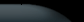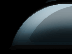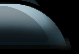###Author Topic: SimpleDice  (Read 2705 times)

0 Members and 1 Guest are viewing this topic.

####F£¥è®##### SimpleDice
« on: January 23, 2009, 11:15:01 pm »
Not posted anything here for a while,had a spare hour so thought I'd write a quick script.
Code: [Select]
`<config><command type = "script"><in>!game</in><out condition = "1" lvalue = "\$dicegame\$" rvalue = "1">#c7#Sorry the game is already in play</out><out type = "break" condition = "1" lvalue = "\$dicegame\$" rvalue = "1"/><out type = "push" extdata = "dicegame">1</out><out type = "push" extdata = "player">%NAME%</out><out type = "push" extdata = "dice"> </out><out type = "push" extdata = "dice2"> </out><out type = "push" extdata = "dice3"> </out><out type = "push" extdata = "dice4"> </out><out type = "push" extdata = "dice5"> </out><out type = "push" extdata = "dice6"> </out><out type = "push" extdata = "dice7"> </out><out type = "push" extdata = "dicenumused">""</out><out type = "push" extdata = "Lose">Lose</out><out type = "push" extdata = "Double">Double</out><out type = "push" extdata = "Treble">Treble</out><out type = "push" extdata = "Points">Points</out><out type = "push" extdata = "Give">Give</out><out>#c5#Type !throwdice to play #c16#or !exp for the rules</out></command><command type = "script" users = "_BOT_SELF_TRIGGER_;\$player\$;"><in>!throwdice</in><out condition = "0" lvalue = "\$dicegame\$" rvalue = "1">#c16#You must type #c7#!game #c16#to play</out><out type = "break" condition = "0" lvalue = "\$dicegame\$" rvalue = "1"/><out type = "push" extdata = "botgo"><operator type = "+" lvalue = "\$botgo\$" rvalue = "1"/></out><out type = "push" extdata = "loop">0</out><out type = "push" extdata = "loop"><operator type = "+" lvalue = "\$loop\$" rvalue ="1"/></out><out type = "push" extdata = "dicenum"><operator type = "+" lvalue = "%RANDOMNUM%" rvalue = "1"/></out><out type = "push" extdata = "dice" condition = "1" lvalue = "\$loop\$" rvalue = "1">\$dicenum\$</out><out type = "push" extdata = "dice2" condition = "1" lvalue = "\$loop\$" rvalue = "2">\$dicenum\$</out><out type = "push" extdata = "dice3" condition = "1" lvalue = "\$loop\$" rvalue = "3">\$dicenum\$</out><out type = "push" extdata = "dice4" condition = "1" lvalue = "\$loop\$" rvalue = "4">\$dicenum\$</out><out type = "goto" extdata = "-6"condition = "0" lvalue = "\$loop\$" rvalue = "4"/><out type = "push" extdata = "loop">0</out><out type = "push" extdata = "loop"><operator type = "+" lvalue = "\$loop\$" rvalue ="1"/></out><out type = "push" extdata = "dicenum"><operator type = "+" lvalue = "%RANDOMNUM%" rvalue = "1"/></out><out type = "push" extdata = "dice5" condition = "1" lvalue = "\$loop\$" rvalue = "1">\$dicenum\$</out><out type = "push" extdata = "dice6" condition = "1" lvalue = "\$loop\$" rvalue = "2">\$dicenum\$</out><out type = "goto" extdata = "-4"condition = "0" lvalue = "\$loop\$" rvalue = "2"/><out type = "push" extdata = "dicetext" condition = "1" lvalue = "\$dice5\$" rvalue = "1">\$Lose\$</out><out type = "push" extdata = "dicetext" condition = "1" lvalue = "\$dice5\$" rvalue = "2">\$Double\$</out><out type = "push" extdata = "dicetext" condition = "1" lvalue = "\$dice5\$" rvalue = "3">\$Points\$</out><out type = "push" extdata = "dicetext" condition = "1" lvalue = "\$dice5\$" rvalue = "4">\$Points\$</out><out type = "push" extdata = "dicetext" condition = "1" lvalue = "\$dice5\$" rvalue = "5">\$Double\$</out><out type = "push" extdata = "dicetext" condition = "1" lvalue = "\$dice5\$" rvalue = "6">\$Lose\$</out><out type = "push" extdata = "dicetext2" condition = "1" lvalue = "\$dice6\$" rvalue = "1">\$Treble\$</out><out type = "push" extdata = "dicetext2" condition = "1" lvalue = "\$dice6\$" rvalue = "2">\$Give\$</out><out type = "push" extdata = "dicetext2" condition = "1" lvalue = "\$dice6\$" rvalue = "3">\$Points\$</out><out type = "push" extdata = "dicetext2" condition = "1" lvalue = "\$dice6\$" rvalue = "4">\$Points\$</out><out type = "push" extdata = "dicetext2" condition = "1" lvalue = "\$dice6\$" rvalue = "5">\$Give\$</out><out type = "push" extdata = "dicetext2" condition = "1" lvalue = "\$dice6\$" rvalue = "6">\$Treble\$</out><out type = "push" extdata = "dicetotal">\$dice\$</out><out type = "push" extdata = "dicetotal"><operator type = "+" lvalue = "\$dicetotal\$" rvalue = "\$dice2\$"/></out><out type = "push" extdata = "dicetotal"><operator type = "+" lvalue = "\$dicetotal\$" rvalue = "\$dice3\$"/></out><out type = "push" extdata = "dicetotal"><operator type = "+" lvalue = "\$dicetotal\$" rvalue = "\$dice4\$"/></out><out type = "self">!checkstatus</out></command><command type = "script" users = "_BOT_SELF_TRIGGER_"><in>!checkstatus</in><out type = "goto" extdata = "12" condition = "1" lvalue = "\$botgo\$" rvalue = "2"/><out type = "push" extdata = "playerdiceholder" condition = "1" lvalue = "\$dicetext\$\$dicetext2\$" rvalue = "\$Double\$\$Points\$"><operator type = "*" lvalue = "\$dicetotal\$" rvalue = "2"/></out><out type = "goto" extdata = "7" condition = "1" lvalue = "\$dicetext\$\$dicetext2\$" rvalue = "\$Double\$\$Points\$"/><out type = "push" extdata = "playerdiceholder" condition = "1" lvalue = "\$dicetext\$\$dicetext2\$" rvalue = "\$Points\$\$Treble\$"><operator type = "*" lvalue = "\$dicetotal\$" rvalue = "3"/></out><out type = "goto" extdata = "5" condition = "1" lvalue = "\$dicetext\$\$dicetext2\$" rvalue = "\$Points\$\$Treble\$"/><out type = "push" extdata = "botdicetotal" condition = "1" lvalue = "\$dicetext\$\$dicetext2\$" rvalue = "\$Points\$\$Give\$"><operator type = "+" lvalue = "\$botdicetotal\$" rvalue = "\$dicetotal\$"/></out><out type = "goto" extdata = "4" condition = "1" lvalue = "\$dicetext\$\$dicetext2\$" rvalue = "\$Points\$\$Give\$"/><out type = "goto" extdata = "3" condition = "1" lvalue = "\$dicetext\$\$dicetext2\$" rvalue = "\$Lose\$\$Points\$"/><out type = "push" extdata = "playerdiceholder">\$dicetotal\$</out><out type = "push" extdata = "playerdicetotal"><operator type = "+" lvalue = "\$playerdicetotal\$" rvalue = "\$playerdiceholder\$"/></out><out type = "self" condition = "1" lvalue = "\$botgo\$" rvalue = "1">!showdice</out><out type = "break"/><out type = "push" extdata = "botdiceholder" condition = "1" lvalue = "\$dicetext\$\$dicetext2\$" rvalue = "\$Double\$\$Points\$"><operator type = "*" lvalue = "\$dicetotal\$" rvalue = "2"/></out><out type = "goto" extdata = "7" condition = "1" lvalue = "\$dicetext\$\$dicetext2\$" rvalue = "\$Double\$\$Points\$"/><out type = "push" extdata = "botdiceholder" condition = "1" lvalue = "\$dicetext\$\$dicetext2\$" rvalue = "\$Points\$\$Treble\$"><operator type = "*" lvalue = "\$dicetotal\$" rvalue = "3"/></out><out type = "goto" extdata = "5" condition = "1" lvalue = "\$dicetext\$\$dicetext2\$" rvalue = "\$Points\$\$Treble\$"/><out type = "push" extdata = "playerdicetotal" condition = "1" lvalue = "\$dicetext\$\$dicetext2\$" rvalue = "\$Points\$\$Give\$"><operator type = "+" lvalue = "\$playerdicetotal\$" rvalue = "\$dicetotal\$"/></out><out type = "goto" extdata = "4" condition = "1" lvalue = "\$dicetext\$\$dicetext2\$" rvalue = "\$Points\$\$Give\$"/><out type = "goto" extdata = "3" condition = "1" lvalue = "\$dicetext\$\$dicetext2\$" rvalue = "\$Lose\$\$Points\$"/><out type = "push" extdata = "botdiceholder">\$dicetotal\$</out><out type = "push" extdata = "botdicetotal"><operator type = "+" lvalue = "\$botdicetotal\$" rvalue = "\$botdiceholder\$"/></out><out type = "self" condition = "1" lvalue = "\$botgo\$" rvalue = "2">!showdice</out></command><command type = "script" users = "_BOT_SELF_TRIGGER_"><in>!showdice</in><out type = "push" extdata = "botgo" condition = "1" lvalue = "\$botgo\$" rvalue = "2">0</out><out> </out><out>                         #c20#[#c1#\$dicetext\$#c20#]    [#c1#\$dicetext2\$#c20#]</out><out>     #c20#[#c1#\$dice\$#c20#]  [#c1#\$dice2\$#c20#]  [#c1#\$dice3\$#c20#]  [#c1#\$dice4\$#c20#]    #c7#Playerscore #c8#= #c20#[#c1#\$playerdicetotal\$#c20#]   #c8#Botscore #c7#= #c20#[#c1#\$botdicetotal\$#c20#]</out><out> </out><out condition = "3" lvalue = "\$playerdicetotal\$" rvalue = "99">#c8#Well done \$player\$ you have beaten the Bot.</out><out type = "push" extdata = "winner" condition = "3" lvalue = "\$playerdicetotal\$" rvalue = "99">1</out><out type = "goto" extdata = "3" condition = "3" lvalue = "\$playerdicetotal\$" rvalue = "99"/><out condition = "3" lvalue = "\$botdicetotal\$" rvalue = "99">#c8#Hard luck \$player\$ you have lost to the Bot.</out><out type = "push" extdata = "winner" condition = "3" lvalue = "\$botdicetotal\$" rvalue = "99">1</out><out type = "self" condition = "1" lvalue = "\$winner\$" rvalue = "1">!win</out><out type = "break" condition = "1" lvalue = "\$winner\$" rvalue = "1"/><out condition = "1" lvalue = "\$botgo\$" rvalue = "1">#c16#The Bot is now throwing the dice</out><out delay = "2000" type = "self" condition = "1" lvalue = "\$botgo\$" rvalue = "1">!throwdice</out><out delay = "2000" condition = "1" lvalue = "\$botgo\$" rvalue = "0">#c16#Please type !throwdice</out></command><command type = "script" users = "_BOT_SELF_TRIGGER_;\$player\$;"><in>!win</in><out type = "pop" extdata = "playerdiceholder"/><out type = "pop" extdata = "botdiceholder"/><out type = "pop" extdata = "dicegame"/><out type = "pop" extdata = "botgo"/><out type = "pop" extdata = "playerdicetotal"/><out type = "pop" extdata = "botdicetotal"/><out type = "pop" extdata = "winner"/><out>&nbsp;&nbsp;&nbsp;&nbsp;&nbsp;&nbsp;&nbsp;&nbsp;#c21#SimpleDice&nbsp;&nbsp;&nbsp;&nbsp;By #c2#F£#c10#¥#c2#è®</out></command><command type = "script"><in>!exp</in><out>#c16#This is a very easy points scoring game against the bot,the first to 100 or more is the winner.</out><out>#c16#If the 2 text dice contain the words Double Points, Points Treble, Points Give or Lose Points then that action is performed.</out><out>#c8#Type !throwdice to play.</out></command> </config>`
OS Microsoft® Windows Vista™ Home Premium, Processor Intel(R) Core(TM)2 Quad CPU  Q8200  @ 2.33GHz, 2331 Mhz, 4 Core(s), 4 Logical Processor(s), Installed Physical Memory (RAM) 6.00 GB